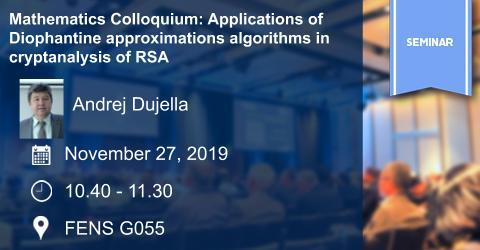## Mathematics Colloquium: Applications of Diophantine approximations alg..

### Mathematics Colloquium: Applications of Diophantine approximations alg..Speaker: Andrej Dujella, University of Zagreb

Title: Applications of Diophantine approximations algorithms in cryptanalysis of RSA

Date/Time: November 27, 2019  /  10.40-11.30

Place: FENS G055

Abstract: To speed up the RSA decryption one may try to use small secret decryption exponent d. The choice of a small d is especially interesting when there is a large difference in computing power between two communicating devices. However, in 1990, Wiener showed that if d < n^(1/4), where n = pq is the modulus of the cryptosystem, then there exist a polynomial time attack on the RSA. He showed that d is the denominator of some convergent p_m/q_m of the continued fraction expansion of e/n, and therefore d can be computed efficiently from the public key (n,e). In this talk, we will discuss similar attacks on RSA and its variants which use results and algorithms from Diophantine approximations, such as Worley's extension of the classical Legendre's theorem on continued fractions and LLL-algorithm for computing short vectors in lattices.

BIO:  Andrej Dujella is professor at the University of Zagreb and Fellow of the Croatian Academy of Sciences and Arts. He received a PhD in mathematics from the University of Zagreb in 1996 and Doctor Honoris Causa of University of Debrecen in 2017. His research interests include Diophantine equations, elliptic curves, polynomial root separation, and applications of Diophantine approximation to cryptography.

Contact: Michel Lavrauw